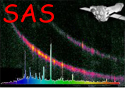XMM-Newton Science Analysis System

epchain (epchain-8.82.1) [xmmsas_20211130_0941-20.0.0]

## Examples

Let us assume we have an observation 0084_0099280101 with three exposures, one TIMING mode exposure PNS008, and two imaging exposures PNS010 (in Small Window Mode) and PNS018 (in Full Frame Mode). Here follow some typical calls to epchain:

```  epchain
```
This processes the first IMAGING mode exposure (i.e. PNS010) and is equivalent to one of the calls
```
epchain datamode=IMAGING odfaccess=odf exposure=010 schedule=S
epchain datamode=IMAGING odfaccess=oal exposure=1
epchain datamode=IMAGING exposure=1
epchain odfaccess=name odf=0084\_0099280101\_PNS01000AUX.FIT
```
If you are interested in the second IMAGING exposure then you may enter
```
epchain exposure=2
```
or (if you like it more verbose) any one of these:
```  epchain datamode=IMAGING odfaccess=odf exposure=018 schedule=S
epchain datamode=IMAGING odfaccess=oal exposure=2
epchain odfaccess=name odf=0084_0099280101_PNS01800AUX.FIT
```
Finally, the TIMING mode exposure (if existent) is processed via any of the calls
```  epchain datamode=TIMING
epchain datamode=TIMING exposure=1
epchain datamode=TIMING odfaccess=oal exposure=1
epchain datamode=TIMING odfaccess=odf exposure=008
epchain datamode=TIMING odfaccess=odf exposure=008 schedule=Y
epchain odfaccess=name odf=0084_0099280101_PNS00800AUX.FIT
```
The commands
```  epchain odfaccess=all
epchain datamode=IMAGING odfaccess=all
```
will process all IMAGING mode exposures in the selected ODF in one call to epchain. All TIMING mode or BURST mode exposures (if available) may be selected accordingly. The call
``` epchain datamode=ALL odfaccess=all
```
will process all exposures of an ODF, independent of observing mode. Sometimes one is interested just in particular (e.g. central) CCDs:
```  epchain ccds=1,4,7,10 exposure=2 ...
```
or one does not want to search for bad pixels (e.g. for short exposures) and no background lightcurve:
```  epchain runbadpix=N getnewbadpix=N runbackground=N ...
```
To create the output filenames according to the ODF (i.e. P0099280101PNS010*.FIT) use the parameter switch (this is the default in the meanwhile)
```  epchain usecanonicalnames=Y ...
```
Finally, the next example shows how to create a “normal” events file together with an “out-of-time” events file by re-using intermediate files (the order of the two calls to epchain is relevant):
```  epchain runbackground=N keepintermediate=raw withoutoftime=Y
```

Some special settings that may be useful for particular observations:
If you have a very soft source and you want to have also the very softest photons to be included in the final event list (e.g. lower threshold 100 eV instead of 150 eV) then (be prepared for a very large event file):

```  epchain screenlowthresh=0 ...  # default: 150
```
If you have an offset pointing in TIMING or BURST mode (either intentionally or because the sky position of the source was not accurately known at the time of the scheduling e.g. for an “Target of Opportunity”) then the correct source position can be entered via parameters (see also Sect. 4) to ensure correct timing and CTI corrections:
```  epchain ... withsrcccords=Y srcra=83.633208 srcdec=22.0124194
```
If you are interested in diagnostic images and plots and the EPEA rejection setup
```  epchain ... witheventmap=Y withphotonmap=Y withpatplot=Y showpah=Y
```

XMM-Newton SOC -- 2021-11-30